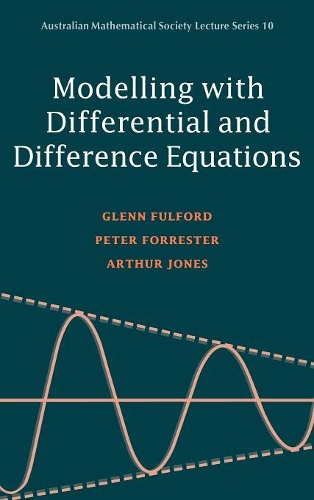•# Modelling with Differential and Difference Equations - Australian Mathematical Society Lecture Series (Hardback)

(author), (author), (author)
£105.00
Hardback 416 Pages / Published: 12/06/1997
• We can order this

Usually dispatched within 3 weeks

The real world can be modelled using mathematics, and the construction of such models is the theme of this book. The authors concentrate on the techniques used to set up mathematical models and describe many systems in full detail, covering both differential and difference equations in depth. Amongst the broad spectrum of topics studied in this book are: mechanics, genetics, thermal physics, economics and population studies. Any student wishing to solve problems via mathematical modelling will find that this book provides an excellent introduction to the subject.

Publisher: Cambridge University Press
ISBN: 9780521440691
Number of pages: 416
Weight: 780 g
Dimensions: 229 x 152 x 27 mm

MEDIA REVIEWS
"...highly recommended...a demanding text; the exercises are excellent and challenging, and some are at the level of research problems. Applied mathematicians, engineers, and scientists will appreciate this book." G.J.F. Junevicus, Choice
"If you are looking for a textbook for an introdutory course on mathematical modeling, with an emphasis on differential and difference equations and basic calculus as the only prerequisite, then give this book serious consideration. It is very well written and very well structured.... overall presentation of material is excellent.... an excellent reference for anyone who has an interest in mathematical modeling....The foundation that this text helps to build will easily allow the reader to move on to more advanced texts on mathematical modeling." The UMAP Journal